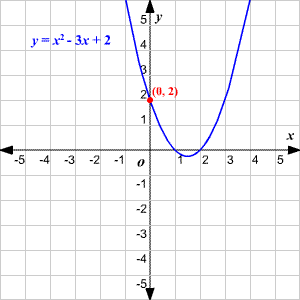# $y$ -Intercepts

The $y$ -intercept of a function is the point(s) where the graph of the function crosses the $y$ -axis.

For most functions, the $y$ -intercept can usually be easily calculated by substituting $0$ for $x$ .

Example:

Find the $y$ -intercept of the parabola

$y={x}^{2}-3x+2$ .

Substitute $0$ for $x$ .

$y={0}^{2}-3\left(0\right)+2$

$y=2$